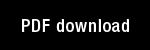Fuzzy lattice neurocomputing (FLN) models

Author(s): Kaburlasos, V. | Petridis, V. |

Year: 2000

Citation: NEURAL NETWORKS Volume: 13 Issue: 10 Pages: 1145-1170

Abstract: In this work it is shown how fuzzy lattice neurocomputing (FLN) emerges as a connectionist paradigm in the framework of fuzzy lattices (FL-framework) whose advantages include the capacity to deal rigorously with: disparate types of data such as numeric and linguistic data, intervals of values, missing and don t care data. A novel notation for the FL-framework is introduced here in order to simplify mathematical expressions without losing content. Two concrete FLN models are presented, namely sigma -FLN for competitive clustering, and FLN with tightest fits (FLNtf) for supervised clustering. Learning by the sigma -FLN, is rapid as it requires a single pass through the data, whereas learning by the FLNtf, is incremental, data order independent, polynomial O(n(3)), and it guarantees maximization of the degree of inclusion of an input in a learned class as explained in the text. Convenient geometric interpretations are provided. The sigma -FLN is presented here as fuzzy-ART s extension in the FL-framework such that sigma -FLN widens fuzzy-ART s domain of application to (mathematical) lattices by augmenting the scope of both of fuzzy ART s choice (Weber) and match functions, and by enhancing fuzzy-ART s complement coding technique. The FLNtf neural model is applied to four benchmark data sets of various sizes for pattern recognition and rule extraction. The benchmark data sets in question involve jointly numeric and nominal data with missing and/or don t care attribute values, whereas the lattices involved include the unit-hypercube, a probability space, and a Boolean algebra. The potential of the FL-framework in computing is also delineated.

Topics: Machine Learning, Models: ART 2 / Fuzzy ART, Modified ART,